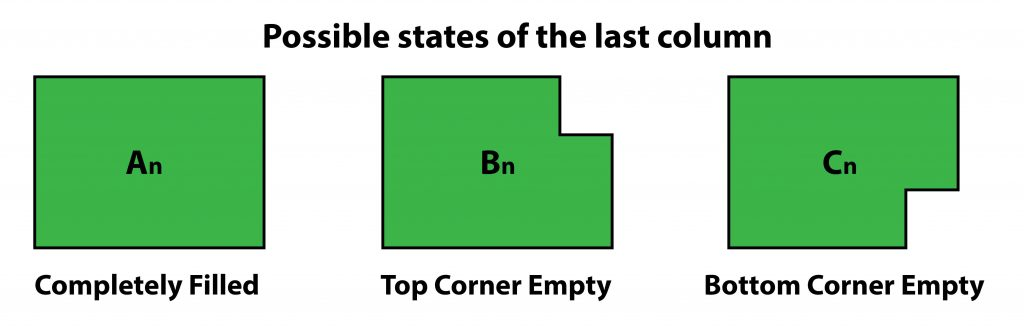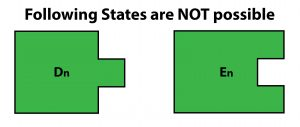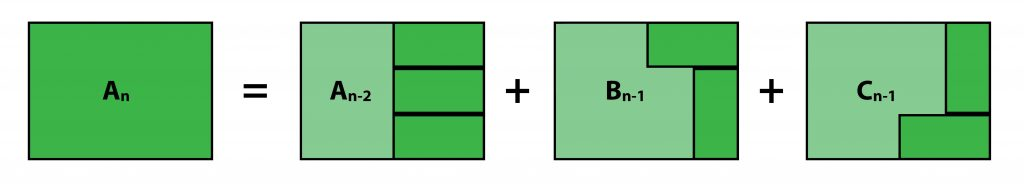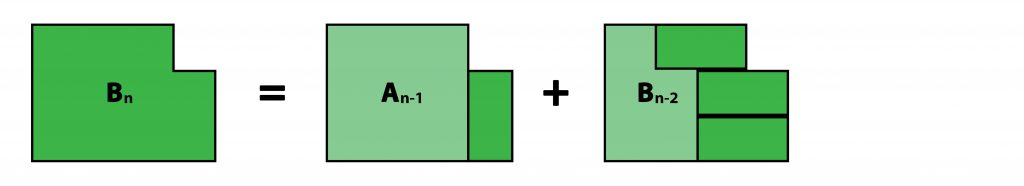# Tiling with Dominoes

Given a 3 x n board, find the number of ways to fill it with 2 x 1 dominoes.

Example 1
Following are all the 3 possible ways to fill up a 3 x 2 board.Example 2
Here is one possible way of filling a 3 x 8 board. You have to find all the possible ways to do so.Examples :

Input : 2
Output : 3

Input : 8
Output : 153

Input : 12
Output : 2131


## Recommended: Please try your approach on {IDE} first, before moving on to the solution.

Defining Subproblems:
At any point while filling the board, there are three possible states that the last column can be in:An =  No. of ways to completely fill a 3 x n board. (We need to find this)
Bn =  No. of ways to fill a 3 x n board with top corner in last column not filled.
Cn =  No. of ways to fill a 3 x n board with bottom corner in last column not filled.


Note: The following states are impossible to reach:Finding Reccurences
Note: Even though Bn and Cn are different states, they will be equal for same ‘n’. i.e Bn = Cn
Hence, we only need to calculate one of them.

Calculating An:Calculating Bn:Final Recursive Relations are:Base Cases:## C++

 // C++ program to find no. of ways  // to fill a 3xn board with 2x1 dominoes.  #include  using namespace std;     int countWays(int n)  {      int A[n + 1], B[n + 1];      A = 1, A = 0, B = 0, B = 1;      for (int i = 2; i <= n; i++) {          A[i] = A[i - 2] + 2 * B[i - 1];          B[i] = A[i - 1] + B[i - 2];      }         return A[n];  }     int main()  {      int n = 8;      cout << countWays(n);      return 0;  }

## Java

 // Java program to find no. of ways  // to fill a 3xn board with 2x1 dominoes.  import java.io.*;     class GFG {         static int countWays(int n)      {          int []A = new int[n+1];          int []B = new int[n+1];          A = 1; A = 0;          B = 0; B = 1;          for (int i = 2; i <= n; i++)           {              A[i] = A[i - 2] + 2 * B[i - 1];              B[i] = A[i - 1] + B[i - 2];          }                 return A[n];      }         // Driver code      public static void main (String[] args)       {          int n = 8;          System.out.println(countWays(n));      }  }     // This code is contributed by anuj_67.

## Python 3

 # Python 3 program to find no. of ways  # to fill a 3xn board with 2x1 dominoes.     def countWays(n):         A =  * (n + 1)      B =  * (n + 1)      A = 1     A = 0     B = 0     B = 1     for i in range(2, n+1):          A[i] = A[i - 2] + 2 * B[i - 1]          B[i] = A[i - 1] + B[i - 2]             return A[n]     n = 8 print(countWays(n))     # This code is contributed by Smitha

## C#

 // C# program to find no. of ways  // to fill a 3xn board with 2x1 dominoes.  using System;     class GFG {         static int countWays(int n)      {          int []A = new int[n+1];          int []B = new int[n+1];          A = 1; A = 0;          B = 0; B = 1;          for (int i = 2; i <= n; i++)           {              A[i] = A[i - 2] + 2 * B[i - 1];              B[i] = A[i - 1] + B[i - 2];          }                 return A[n];      }         // Driver code      public static void Main ()       {          int n = 8;          Console.WriteLine(countWays(n));      }  }     // This code is contributed by anuj_67.

## PHP

 

Output :

153My Personal Notes arrow_drop_upCheck out this Author's contributed articles.

If you like GeeksforGeeks and would like to contribute, you can also write an article using contribute.geeksforgeeks.org or mail your article to contribute@geeksforgeeks.org. See your article appearing on the GeeksforGeeks main page and help other Geeks.

Please Improve this article if you find anything incorrect by clicking on the "Improve Article" button below.

Improved By : vt_m, Smitha Dinesh Semwal